# Concept of-complex-frequency

18 de Apr de 2012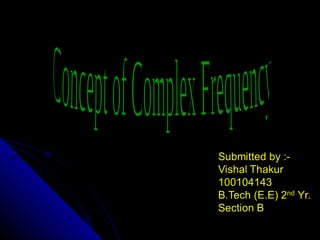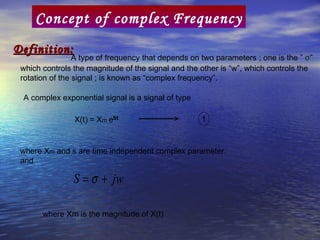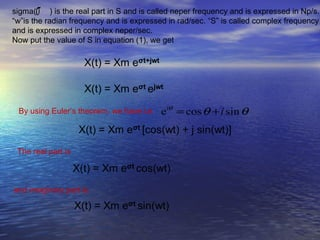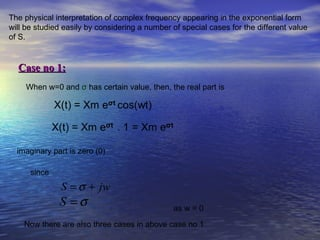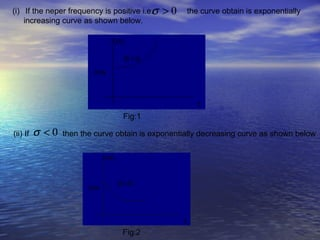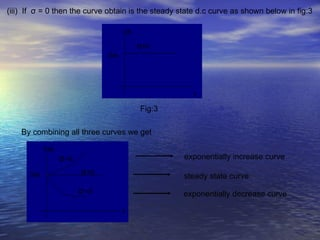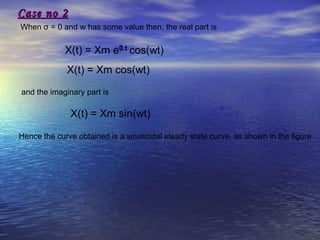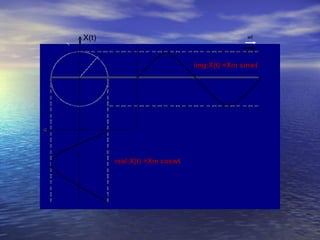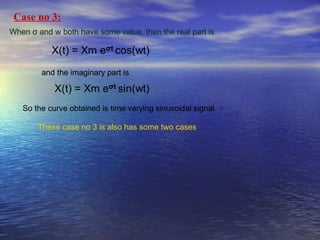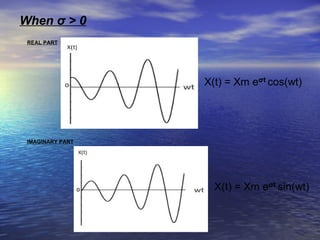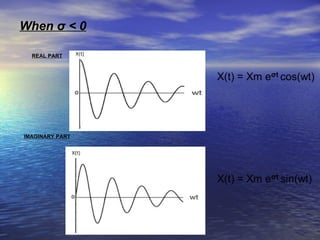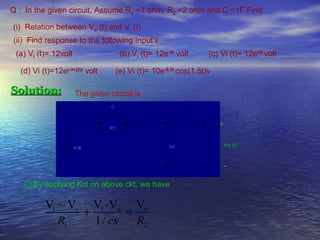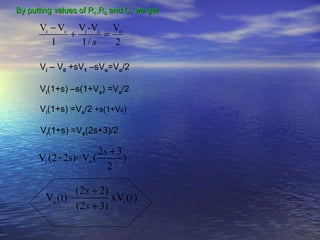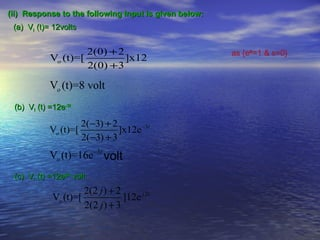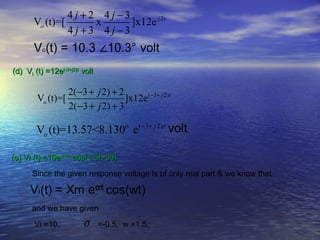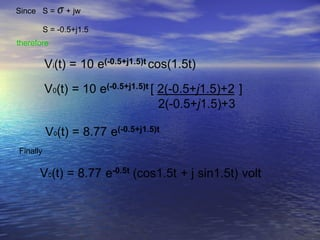1 de 17

### Concept of-complex-frequency

1. Concept of complex Frequency Definition: A type of frequency that depends on two parameters ; one is the ” σ” which controls the magnitude of the signal and the other is “w”, which controls the rotation of the signal ; is known as “complex frequency”. A complex exponential signal is a signal of type 1 where Xm and s are time independent complex parameter. and S = σ + jw where Xm is the magnitude of X(t)
2. sigma(σ ) is the real part in S and is called neper frequency and is expressed in Np/s. “w”is the radian frequency and is expressed in rad/sec. “S” is called complex frequency and is expressed in complex neper/sec. Now put the value of S in equation (1), we get By using Euler’s theorem. we have i.e eiθ = cos θ + i sin θ The real part is and imaginary part is
3. The physical interpretation of complex frequency appearing in the exponential form will be studied easily by considering a number of special cases for the different value of S. Case no 1: When w=0 and σ has certain value, then, the real part is imaginary part is zero (0) since S = σ + jw S =σ as w = 0 Now there are also three cases in above case no 1
4. σ (i) If the neper frequency is positive i.e. >0 the curve obtain is exponentially increasing curve as shown below. Fig:1 (ii) If σ <0 then the curve obtain is exponentially decreasing curve as shown below Fig:2
5. (iii) If σ = 0 then the curve obtain is the steady state d.c curve as shown below in fig:3 Fig:3 By combining all three curves we get exponentially increase curve steady state curve exponentially decrease curve
6. Case no 2 When σ = 0 and w has some value then, the real part is and the imaginary part is Hence the curve obtained is a sinusoidal steady state curve, as shown in the figure
7. X(t) wt wt img:X(t) =Xm sinwt real:X(t) =Xm coswt
8. Case no 3: When σ and w both have some value, then the real part is and the imaginary part is So the curve obtained is time varying sinusoidal signal These case no 3 is also has some two cases
9. When σ > 0 REAL PART IMAGINARY PART
10. When σ < 0 REAL PART IMAGINARY PART
11. Q : In the given circuit, Assume R1 =1 ohm, R2 =2 ohm and C =1F Find (i) Relation between Vo (t) and Vi (t) (ii) Find response to the following Input’s (a) Vi (t)= 12volt (b) Vi (t)= 12e-3t volt (c) Vi (t)= 12ei2t volt (d) Vi (t)=12e(-3+j2)t volt (e) Vi (t)= 10e-0.5t cos(1.5t)v Solution: The given circuit is + Vo (t) - (i) By applying Kcl on above ckt, we have Vi − Vo Vi -Vo Vo + = R1 1 / cs R2
12. By putting values of R1,R2 and C, we get Vi − Vo Vi -Vo Vo + = 1 1/ s 2 Vi – V0 +sV1 –sVo=Vo/2 Vi(1+s) –s(1+Vo) =Vo/2 Vi(1+s) =Vo/2 +s(1+Vo) Vi(1+s) =Vo(2s+3)/2 2s + 3 Vi (2+2s)=V0 ( ) 2 (2 s + 2) Vo (t)= xVi (t ) (2 s + 3)
13. (ii) Response to the following Input is given below: (a) Vi (t)= 12volts 2(0) + 2 Vo (t)=[ ]x12 2(0) + 3 Vo (t)=8 volt (b) Vi (t) =12e-3t 2(−3) + 2 Vo (t)=[ ]x12e −3t 2(−3) + 3 Vo (t)=16e −3t (c) Vi (t) =12ej2t volt 2(2 j ) + 2 Vo (t)=[ ]12e j 2t 2(2 j ) + 3
14. 4 j + 2 4 j −3 Vo (t)=[ x ]x12e j 2t 4 j +3 4 j −3 (d) Vi (t) =12e(-3+j2)t volt 2(−3 + j 2) + 2 Vo (t)=[ ]x12e ( −3+ j 2)t 2(−3 + j 2) + 3 Vo (t)=13.57<8.130o e( −3+ j 2)t (e) Vi (t) =10e-0.5t cos(1.5t+30) Since the given response voltage is of only real part & we know that and we have given Vi =10, σ =-0.5, w =1.5,
15. Since S = σ + jw S = -0.5+j1.5 therefore Finally